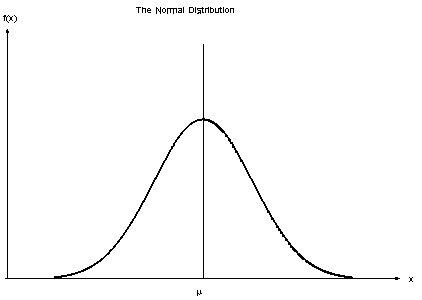Quantitative Data Analysis – Normal and Skewed Distributions

(1) Normal Distribution:

Classic bell shaped curve, it is the predicted distribution when using equally likely sets of results. For example, if a light bulb has a lifetime of 100 hours we would expect some bulbs to last a little longer than 100 hours and some to last a little less.Characteristics of a Normal Distribution:
– The three measures of central tendency, mean, median and mode are all in the exact mid-point (the middle part of the graph/the peak of the curve).
– The distribution is symmetrical.

(2) Skewed Distribution

This occurs when the scores are not equally distributed around the mean.

Positive Skew – The best way to imagine the shape of a positive skew is to think of the scores on a very difficult exam, were few people got a high mark being plotted on a graph. Most of the scores would lie to the left side of the x axis with fewer scores being plotted at the higher end of the x axis (the right).

Revision Tip – When thinking of the shape of a positive skewed distribution, think of the shape of your right foot.

Characteristics of a Positive Skewed Distribution Graph:
– Central tendency order is plotted mode, median followed by the mean.

Negative Skew – The best way to remember the shape of a negative skewed is to imagine the scores on a very easy exam, were few people got a low mark, were plotted on a graph. In this situation, people would obtain a higher score and therefore, most scores would sit to the right side of the x axis with fewer scores sitting to the left side of the x axis.

Revision Tip: When thinking of the shape a of negative skewed distribution, think of the shape of your left foot.

Characteristics of a Negative Skewed Distribution Graph:
– Central tendency order is plotted mean, median followed by the mode.